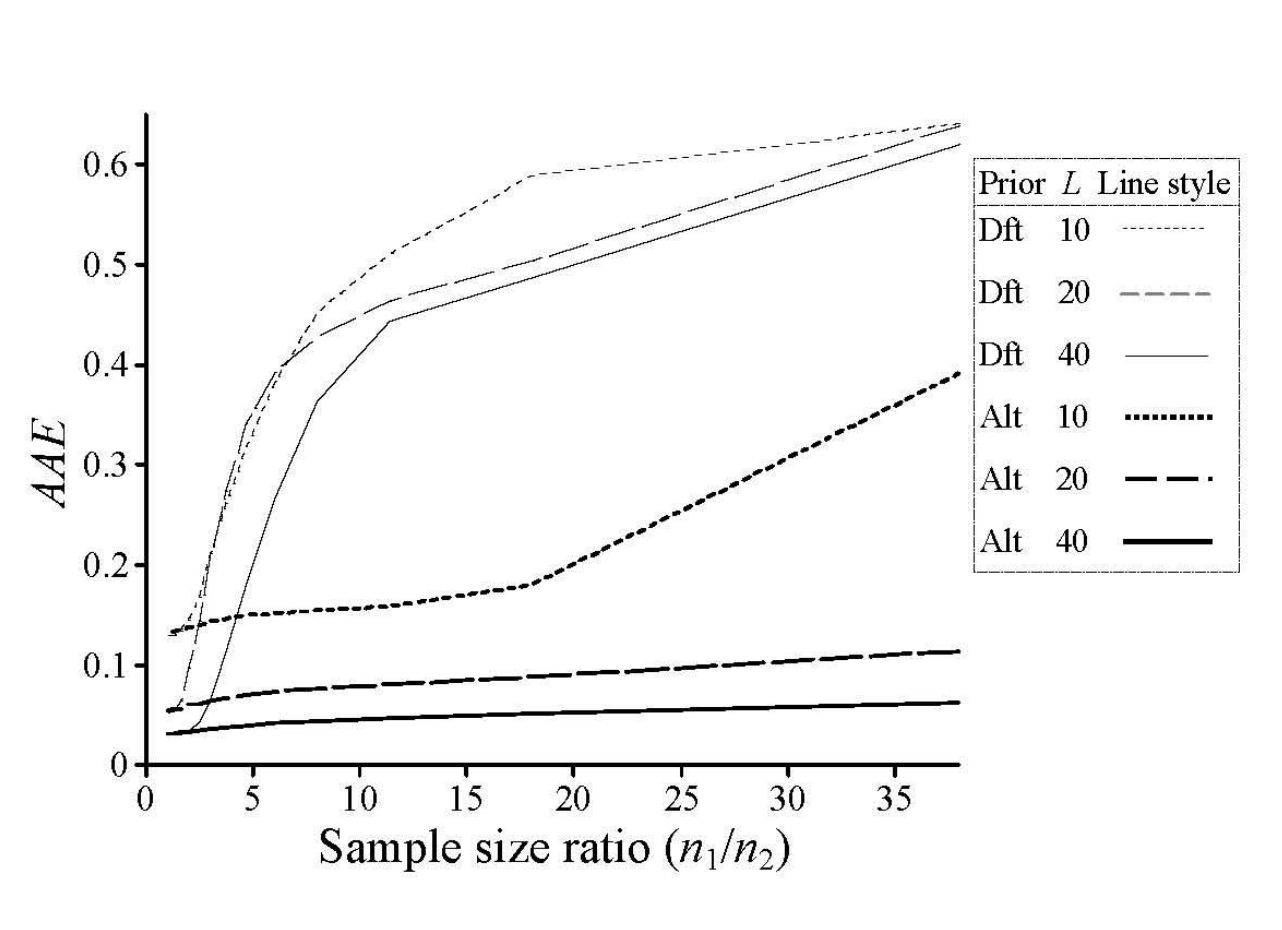# Unbalanced population sampling and STRUCTURE

The utility and intuition offered by the program STRUCTURE, and more generally, the ‘admixture’ model of Pritchard et al. (2000) are unquestioned – with tens of thousands of citations, it retains its lead among the most popular population genetics software. However, much like any method, STRUCTURE comes with its caveats, which recent works have pointed to using simulations and meta-analyses (see here, and also the studies of Gilbert et al. 2012, Evanno et al. 2005, Puechmaille 2016). In a recent manuscript, Wang (2016) quantifies the effect of unbalanced sampling and specification of ancestry priors on STRUCTURE’s estimates.
Ancestry priors (specified by the term ‘alpha’ in STRUCTURE) follows a Dirichlet distribution on the number of ancestral populations (often denoted as ‘K’). STRUCTURE allows two modes of specifying alpha (here a) – it either assumes a uniform prior (i.e. each individual’s multi-locus genotype is assumed to be sampled uniformly from ‘K’ populations with a probability of 1/K), or can be inferred per population (such that a1a2≠…≠aK).
Wang (2017) performed a series of simulations to formally assess the effect of the two ancestry prior specification methods on analyses of unbalanced populations. He varied the number of sampled loci (L = 10, 20, 40, 50), and the number of ancestral populations (K = 3, 6, 12, 24, 48), and levels of differentiation (Fst = 0.05, 0.1, 0.2), and performed long, and 20 replicated runs of STRUCTURE by varying the initial a (set to 1.0 by default). He notes that for greater number of ancestral populations (K), values of a lower than 1.0 are required to deliver accurate inferences. Accuracy was estimated at two levels – in estimation of the number of ancestral populations (as ascertained by the method of Evanno et al. 2005, and Pritchard 2000), and in estimates of admixture proportions (assignment probabilities).Accuracy, measured here as average assignment errors (AAEs), as a function of the extent of unbalanced among populations. Figure 1 from Wang (2016). Dft = default value of alpha = 1.0 in STRUCTURE, Alt = alternative priors, and L quantifies the number of sampled loci. Simulated data comprised of three populations under the island model.

His key findings include (1) inaccurate estimates of both K and assignment probabilities due to unbalanced population sizes while using the default prior of a = 1.0, (2) accurate estimates of assignment probabilities while using the alternative prior (except at large values of K, or in highly unbalanced populations), (3) the estimator of Pritchard et al. (2000) is more accurate in recapitulating ancestral populations than the estimator of Evanno et al. (2005).
This study is important in that it emphasizes the need to perform your STRUCTURE analyses using the alternative prior model of a, rather than using the default value of 1.0. Wang (2016) recommends that you set the initial value of a to be equal to 1/K (i.e. much less than 1.0) for improved accuracy.
Reference:
Wang, J., 2016. The computer program Structure for assigning individuals to populations: easy to use but easier to misuse. Molecular Ecology Resources.  http://dx.doi.org/10.1111/1755-0998.12650

This entry was posted in bioinformatics, genomics, howto, methods, population genetics, software, STRUCTURE and tagged , , . Bookmark the permalink.